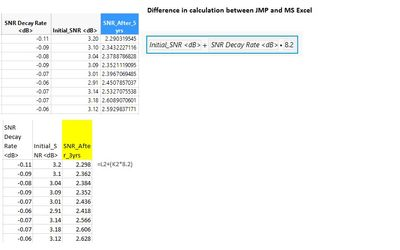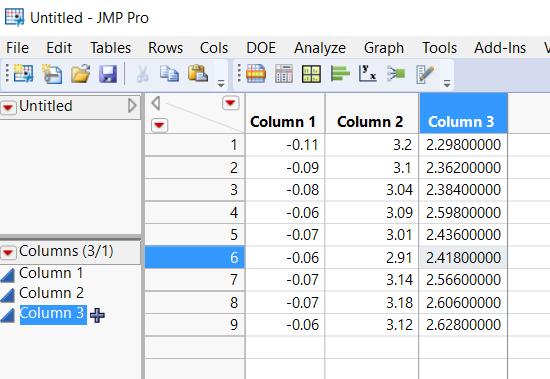Choose Language Hide Translation Bar
Highlighted

## Calculation difference between actual(MS Excel) and JMP

Hi,

Recently I noticed when I am doing a simple calculation using formula there is a delta of 0.0xx in JMP when compare to MS Excel or a normal calculator(refer to attached graphic). Can I know whether this is expected in JMP.

Thanks.1 ACCEPTED SOLUTION

Accepted Solutions
Highlighted

## Re: Calculation difference between actual(MS Excel) and JMP

I have attached the data table I used.  Below is an image of the tableJim
4 REPLIES 4
Highlighted

## Re: Calculation difference between actual(MS Excel) and JMP

Running with JMP 13.2.1 and Office 365 I got the identical values between the calculations in JMP and Excel.

Jim
Highlighted

## Re: Calculation difference between actual(MS Excel) and JMP

Hi Jim,
I guess this could be some sort of "bug" :) I use a normal calculator and the results follow the Excel after all this calculation supposedly gave out 3-decimal point but result in JMP shows up to 9-decimal point.
Highlighted

## Re: Calculation difference between actual(MS Excel) and JMP

I have attached the data table I used.  Below is an image of the tableJim
Highlighted

## Re: Calculation difference between actual(MS Excel) and JMP

I guess this has to do with Col Info > Format. In my JMP data, the first 2 columns were set to Fixed but the result column was set to Best. I think the calculation result actually took the values(contained 9-decimal point) from the 2 columns which was set to Fixed.

That data actually came from a test result so I guess I wasn't aware that it has so many decimal points :-)

Anyway, thanks a lot Jim for your response.

Article Labels

There are no labels assigned to this post.